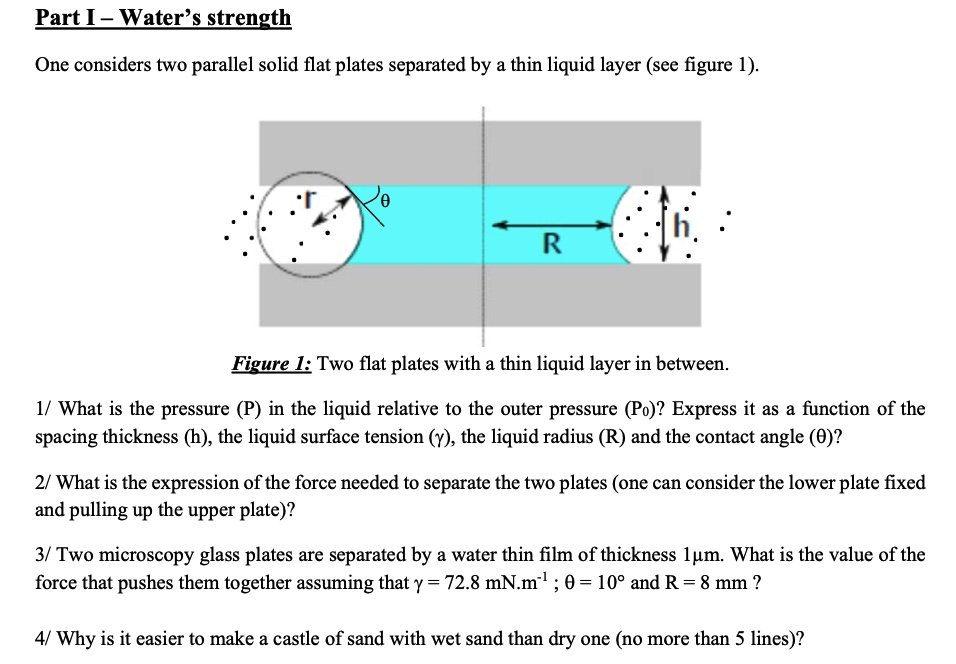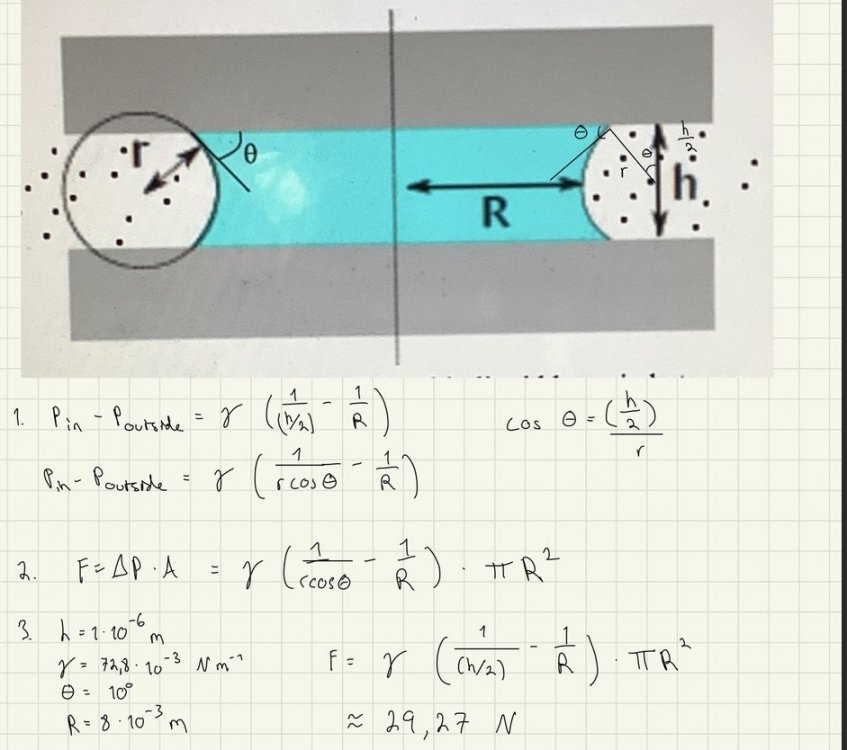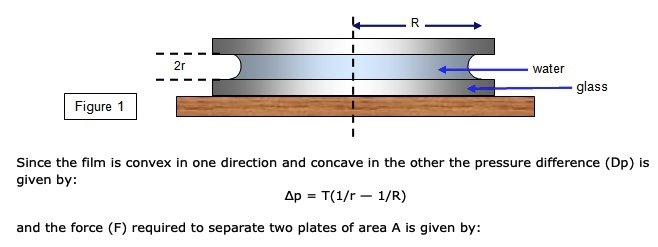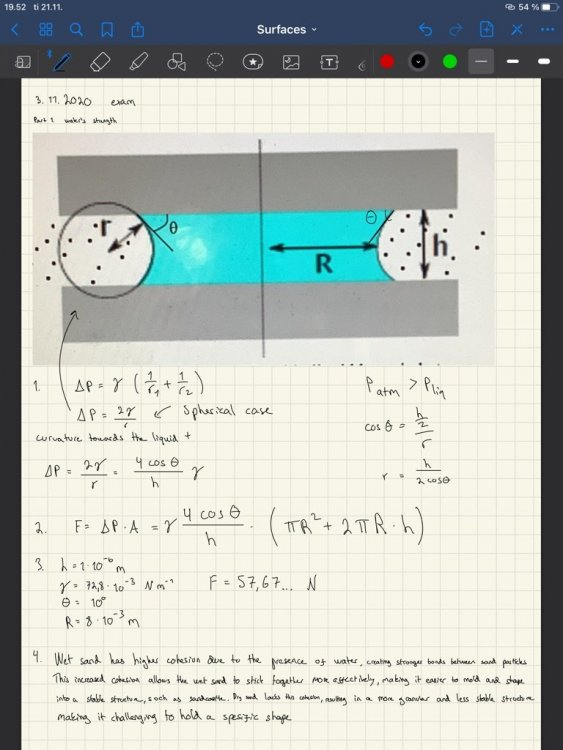# Help to solve this problem!

## Recommended Posts

This problem doesn't seem complicated, but I still can't get it done. I can't figure out how to write the equation with all the required parameters in the first part. I have used the Laplace equation for this one (This is probably correct). Also the third part, How can I calculate the force with knowledge of the dimensions of the plates?##### Share on other sites

33 minutes ago, VilJ said:

This problem doesn't seem complicated, but I still can't get it done. I can't figure out how to write the equation with all the required parameters in the first part. I have used the Laplace equation for this one (This is probably correct). Also the third part, How can I calculate the force with knowledge of the dimensions of the plates?

Is this homework ?

Then it belongs in the homework section.

Hints

1. 1) is the liquid pressure greater than or less than the outside pressure ?  -  Why ?
Then do a force balance between the pressures and the surface tension along the curved interface.
2. It is usual for the liquid to be considered as a circular blob so you can calculate the area
3. Over to you to put the values into your formulae.

##### Share on other sites

31 minutes ago, VilJ said:

I can't figure out how to write the equation with all the required parameters in the first part. I have used the Laplace equation for this one (This is probably correct).

Hint: can you express r in terms of h & theta?

##### Share on other sites

The first thing I did was to express r in terms of h and theta, but then I won't be able to preserve the h?

11 hours ago, studiot said:

Is this homework ?

Then it belongs in the homework section.

Hints

1. 1) is the liquid pressure greater than or less than the outside pressure ?  -  Why ?
Then do a force balance between the pressures and the surface tension along the curved interface.
2. It is usual for the liquid to be considered as a circular blob so you can calculate the area
3. Over to you to put the values into your formulae.

My bad I'm new hereThanks for the help! Ps. This is actually an exam that I'm trying to get done before the real one.

11 hours ago, sethoflagos said:

Hint: can you express r in terms of h & theta?

The first thing I did was to express r in terms of h and theta, but then I won't be able to preserve the h?

##### Share on other sites

Appreciation for the plus marks, but answers to our questions are even more appreciated.

And what about the other questions, What for instance did you get for Seth's suggestion?

Edited by studiot
##### Share on other sites

2 hours ago, studiot said:

Appreciation for the plus marks, but answers to our questions are even more appreciated.

And what about the other questions, What for instance did you get for Seth's suggestion?

This is what I've had for this one... Where am I going wrong?Edited by VilJ
##### Share on other sites

Part 1

You are working in the right direction, but if r = h/2cosθ (which is correct)

then how is 1/r  = 1/(h/2) in your first line ?

##### Share on other sites

15 hours ago, studiot said:

Part 1

You are working in the right direction, but if r = h/2cosθ (which is correct)

then how is 1/r  = 1/(h/2) in your first line ?

So you're telling me that I'm supposed to work with r rather than h/2? I found this example which stated that this problem is supposed to be calculated differently. If I calculate with just r then this is super simple.##### Share on other sites

1 hour ago, VilJ said:

So you're telling me that I'm supposed to work with r rather than h/2? I found this example which stated that this problem is supposed to be calculated differently. If I calculate with just r then this is super simple.

This is a form the Young-Laplace equation as I understand it. Your earlier version with 1/(h/2) instead of 1/r was incorrect for the diagramme given.

But note that the usual form is dP= -T(1/r + 1/R). It only becomes the form quoted above AFTER you have performed algebraic manipulation of the signs. I suggest you apply more focus to distinguishing between the standard forms of equations and expressions lifted from part-completed calculations.

Edited by sethoflagos
Clarification
##### Share on other sites

2 hours ago, VilJ said:

So you're telling me that I'm supposed to work with r rather than h/2? I found this example which stated that this problem is supposed to be calculated differently. If I calculate with just r then this is super simple.

Guiding would be better than telling.

You still haven't answered my questions.

Since you are doing this in preparation for a real exam it is no good just looking up formulae on the net (especially as I suspect it is wrong but I can't see the rest of what you found).

You need to understand what you are doing.

And that is exactly what both Seth and I are trying to help you with.

So let us go back to first principles and answer my simple questions.

Do you have to derive your equations or have you been given them in lectures ?

Using your knowledge of Physics is the internal ressure greater than or less than the external  ?

Why did I suggest you will have a circular blob ?

You new picture is wrong because the vertical meniscus is not a complete semicircle.

Why do you think this is the case ?

##### Share on other sites

8 hours ago, studiot said:

Guiding would be better than telling.

You still haven't answered my questions.

Since you are doing this in preparation for a real exam it is no good just looking up formulae on the net (especially as I suspect it is wrong but I can't see the rest of what you found).

You need to understand what you are doing.

And that is exactly what both Seth and I are trying to help you with.

So let us go back to first principles and answer my simple questions.

Do you have to derive your equations or have you been given them in lectures ?

Using your knowledge of Physics is the internal ressure greater than or less than the external  ?

Why did I suggest you will have a circular blob ?

You new picture is wrong because the vertical meniscus is not a complete semicircle.

Why do you think this is the case ?

Okay, so I did the exercise again and noticed that in this teacher's following exam, he had the same question except he asked us to estimate the water pile as a cylinder. Here is what I've done with a little bit more detail. The answer is exactly the same.##### Share on other sites

Since you seem to just want to copy others, rather than answer my questions I will leave you to it.

##### Share on other sites

On 11/21/2023 at 10:40 PM, studiot said:

Since you seem to just want to copy others, rather than answer my questions I will leave you to it.

That seems totally unnecessary dudeI went through the exercise with the recommended textbook to come up with this solution and put a more detailed answer up here. Idk why you feel the urge to be an asshole about it...

## Create an account

Register a new account